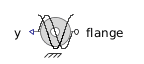DCV 42 CP - MapleSim Help

DCV 42 CP

4-way 2-position close-parallel directional control valveDescription

The DCV 42 CP component is a 4-way 2-position valve. The area between pairs of ports varies as a function of the spool input signal, $s$, and the parameters ${A}_{\mathrm{max}}$, ${A}_{\mathrm{min}}$, and $\mathrm{band}$. The following table gives the areas for two values of the spool input.

 Spool Input P-A B-T 0 ${A}_{\mathrm{min}}$ ${A}_{\mathrm{min}}$ 1 ${A}_{\mathrm{max}}$ ${A}_{\mathrm{max}}$

Transitions are centered at the midpoint, with the width given by the $\mathrm{band}$ parameter, as shown in the following diagram.Formulation Approaches Two approaches were taken for formulation of the flow equation inside the device. When the boolean parameter $\mathrm{Use constant Cd}$ is true, a constant coefficient of discharge (${C}_{d}$) is used, otherwise a variable coefficient of discharge with maximum value (${C}_{d\left(\mathrm{max}\right)}$) and a critical flow number (${\mathrm{Crit}}_{\mathrm{no}}$) are used.Optional Volumes The boolean parameters Use volume A, Use volume B, Use volume P, and Use volume T, when true, add optional volumes ${V}_{A}$, ${V}_{B}$, ${V}_{P}$, and ${V}_{T}$ to ports A, B, P, and T, respectively. See Port Volumes for details. If two orifices or valves are connected, enabling a volume at the common port reduces the stiffness of the system and improves the solvability.Connections

 Name Description Modelica ID $\mathrm{portA}$ Hydraulic port A portA $\mathrm{portB}$ Hydraulic port B portB $\mathrm{portP}$ Hydraulic port P (pump) portP $\mathrm{portT}$ Hydraulic port T (tank) portT $s$ Spool input signal sParametersGeneral

 Name Default Units Description Modelica ID ${A}_{\mathrm{max}}$ $1·{10}^{-4}$ ${m}^{2}$ Maximum orifice opening area; available when use scales is true Amax ${A}_{\mathrm{min}}$ $1·{10}^{-12}$ ${m}^{2}$ Minimum orifice opening area (leakage) Amin $\mathrm{band}$ $0.1$ Transition length in normalized spool displacement units bandOrifice

 Name Default Units Description Modelica ID $\mathrm{Use constant Cd}$ $\mathrm{true}$ True (checked) means a constant coefficient of discharge is implemented, otherwise a variable ${C}_{d}$ is used in flow calculation UseConstantCd ${C}_{d}$ $0.7$ Flow-discharge coefficient; used when $\mathrm{Use constant Cd}$ is true Cd ${\mathrm{\Re }}_{\mathrm{Cr}}$ $12$ Reynolds number at critical flow; used when $\mathrm{Use constant Cd}$ is true ReCr ${C}_{d\left(\mathrm{max}\right)}$ $0.7$ Maximum flow-discharge coefficient; used when $\mathrm{Use constant Cd}$ is false Cd_max ${\mathrm{Crit}}_{\mathrm{no}}$ $1000$ Critical flow number; used when $\mathrm{Use constant Cd}$ is false Crit_noOptional Volumes

X corresponds to ports A, B, P, and T.

 Name Default Units Description Modelica ID $\mathrm{Use volume X}$ $\mathrm{false}$ True (checked) means a hydraulic volume chamber is added to portX useVolumeX ${V}_{X}$ $1·{10}^{-6}$ ${m}^{3}$ Volume of chamber attached to portX Vx